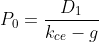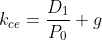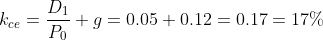Scan QR code or get instant email to install app

Question:

# The following data are reported for Moving Vans, Inc.:Dividend yield 5%Dividend payout 20%Return on equity 15%Assuming Moving Vans' dividend yield, dividend payout, and return on equity will remain constant indefinitely, the cost of equity capital is closest to:

A 17%.
explanation

1212Using the constant growth dividend model, the price for a common stock is:Solving for k :where:
P = current stock price
D = year end expected dividend
k = cost of common equity capital
g = sustainable (constant) growth of equity, earnings, and dividends
The sustainable growth for a company's dividends equal the ROE times the earnings retention rate. The earnings retention rate equals 1 minus the dividend payout rate. Therefore:
g = 0.15 × (1 − 0.20) = 0.12